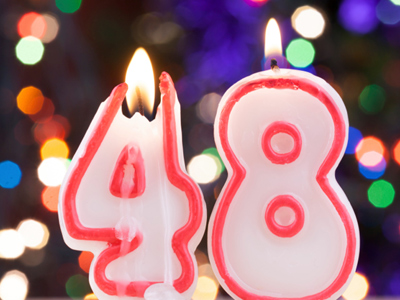What is 48% as a decimal?

# Converting Percentages to Decimals

This Math quiz is called 'Converting Percentages to Decimals' and it has been written by teachers to help you if you are studying the subject at middle school. Playing educational quizzes is a fabulous way to learn if you are in the 6th, 7th or 8th grade - aged 11 to 14.

It costs only \$12.50 per month to play this quiz and over 3,500 others that help you with your school work. You can subscribe on the page at Join Us

Part/Whole = Percent/100

The symbol used to show percent is “%” so 48 percent would be 48%. The “48” is the “part” and the “%” is the whole which means that 48% can also be shown as 48/100.

Converting Percentages to Decimals:

To convert a percentage to a decimal is probably the easiest problem to solve in math. All that is need is division. Looking at the percentage listed above, i.e., 48%, the 48 is divided by the number “100”. The “%” symbol used in percentage numbers always represents the number “100”. So to convert 48% to a decimal you would state the problem as: 48 ÷ 100 = 0.48.

1.
99% = ___
9.09
9.9
.099
.99
Take the percent number 99 and divide it by the % which is the same as 100 as follows: 99 ÷ 100 = .99. Answer (d) is the correct answer
2.
212% = ___
2.12
21.2
.212
.0212
Take the percent number 212 and divide it by the % which is the same as 100 as follows: 212 ÷ 100 = 2.12. Answer (a) is the correct answer
3.
171% = ___
.171
17.1
1.71
10.71
Take the percent number 171 and divide it by the % which is the same as 100 as follows: 171 ÷ 100 = 1.71. Answer (c) is the correct answer
4.
16% = ___
.016
.16
1.6
1.06
Take the percent number 16 and divide it by the % which is the same as 100 as follows: 16 ÷ 100 = .16. Answer (b) is the correct answer
5.
270% = ____
27.0
.27
2.7
.027
Take the percent number 270 and divide it by the % which is the same as 100 as follows: 270 ÷ 100 = 2.7. Answer (c) is the correct answer
6.
349% = ___
3.49
34.9
.349
.035
Take the percent number 349 and divide it by the % which is the same as 100 as follows: 349 ÷ 100 = 3.49. Answer (a) is the correct answer
7.
180% = ____
1.8
.18
18.0
.018
Take the percent number 180 and divide it by the % which is the same as 100 as follows: 180 ÷ 100 = 1.8. Answer (a) is the correct answer
8.
48% = ___
4.8
4.08
.048
.48
Take the percent number 48 and divide it by the % which is the same as 100 as follows: 48 ÷ 100 = .48. Answer (d) is the correct answer
9.
535% = ___
.535
5.35
53.5
.0535
Take the percent number 535 and divide it by the % which is the same as 100 as follows: 535 ÷ 100 = 5.35. Answer (b) is the correct answer
10.
125% = ___
.125
1.25
12.5
.13
Take the percent number 125 and divide it by the % which is the same as 100 as follows: 125 ÷ 100 = 1.25. Answer (b) is the correct answer
Author:  Christine G. Broome